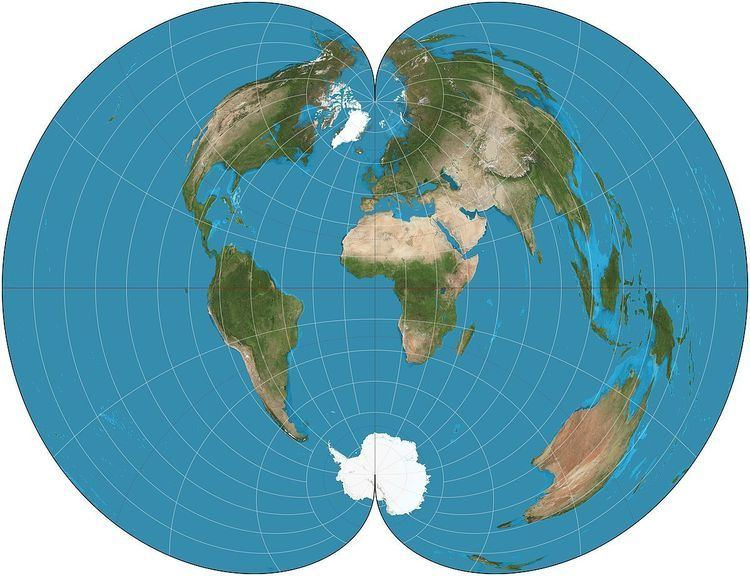# Polyconic projection

Updated on
Covid-19Polyconic can refer either to a class of map projections or to a specific projection known less ambiguously as the American Polyconic. Polyconic as a class refers to those projections whose parallels are all non-concentric circular arcs, except for a straight equator, and the centers of these circles lie along a central axis. This description applies to projections in equatorial aspect.

As a specific projection, the American polyconic projection is conceptualized as "rolling" a cone tangent to the Earth at all parallels of latitude, instead of a single cone as in a normal conic projection. Each parallel is a circular arc of true scale. The scale is also true on the central meridian of the projection. The projection was in common use by many map-making agencies of the United States from the time of its proposal by Ferdinand Rudolph Hassler in 1825 until the middle of the 20th century.

The projection is defined by:

x = cot φ sin ( ( λ λ 0 ) sin φ ) y = φ φ 0 + cot φ ( 1 cos ( ( λ λ 0 ) sin φ ) )

where λ is the longitude of the point to be projected; φ is the latitude of the point to be projected; λ0 is the longitude of the central meridian, and φ0 is the latitude chosen to be the origin at λ0. To avoid division by zero, the formulas above are extended so that if φ0 = 0 then x = λ − λ0 and y = 0.

## References

Similar Topics
The Stooge
Ray Baverstock
Michael Murphy (actor)
Topics